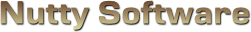Glows

Sorry, it appears you don't have support for WebGL.

In order to run this demo, you must meet the following requirements.

• You have a WebGL compatible video card with the latest drivers.
• Your video card is not blacklisted. You can check the current blacklist on Khronos.

Some browsers may require additional configuration in order to get WebGL to run. If you are having problems running this demo, visit the following sites.

Use mouse.

#### Glow Mode

							
/// <summary>
/// </summary>

/// <summary>
/// Material source structure.
/// <summary>
struct MaterialSource
{
vec3 Ambient;
vec4 Diffuse;
vec3 Specular;
float Shininess;
vec2 TextureOffset;
vec2 TextureScale;
};

/// <summary>
/// Attributes.
/// <summary>
attribute vec3 Vertex;
attribute vec2 Uv;
attribute vec3 Normal;

/// <summary>
/// Uniform variables.
/// <summary>
uniform mat4 ProjectionMatrix;
uniform mat4 ViewMatrix;
uniform mat4 ModelMatrix;
uniform vec3 ModelScale;

uniform MaterialSource Material;

/// <summary>
/// Varying variables.
/// <summary>
varying vec4 vWorldVertex;
varying vec3 vWorldNormal;
varying vec2 vUv;
varying vec3 vViewVec;

/// <summary>
/// <summary>
void main ()
{
// Transform the vertex
vWorldVertex = ModelMatrix * vec4(Vertex * ModelScale, 1.0);
vec4 viewVertex = ViewMatrix * vWorldVertex;
gl_Position = ProjectionMatrix * viewVertex;

// Setup the UV coordinates
vUv = Material.TextureOffset + (Uv * Material.TextureScale);

// Rotate normal
vWorldNormal = normalize(mat3(ModelMatrix) * Normal);

// Calculate view vector (for specular lighting)
vViewVec = normalize(-viewVertex.xyz);
}


							
/// <summary>
/// Vertex shader for rendering a 2D plane on the screen. The plane should be sized
/// from -1.0 to 1.0 in the x and y axis. This shader can be shared amongst multiple
/// </summary>

/// <summary>
/// Attributes.
/// <summary>
attribute vec3 Vertex;
attribute vec2 Uv;

/// <summary>
/// Varying variables.
/// <summary>
varying vec2 vUv;

/// <summary>
/// <summary>
void main ()
{
gl_Position = vec4(Vertex, 1.0);

// Flip y-axis
vUv = Uv;
}


							
/// <summary>
/// </summary>

#ifdef GL_ES
precision highp float;
#endif

/// <summary>
/// Light source structure.
/// <summary>
struct LightSource
{
int Type;
vec3 Position;
vec3 Attenuation;
vec3 Direction;
vec3 Colour;
float OuterCutoff;
float InnerCutoff;
float Exponent;
};

/// <summary>
/// Material source structure.
/// <summary>
struct MaterialSource
{
vec3 Ambient;
vec4 Diffuse;
vec3 Specular;
float Shininess;
vec2 TextureOffset;
vec2 TextureScale;
};

/// <summary>
/// Uniform variables.
/// <summary>
uniform int NumLight;
uniform LightSource Light;
uniform MaterialSource Material;
uniform sampler2D Sample0;

/// <summary>
/// Varying variables.
/// <summary>
varying vec4 vWorldVertex;
varying vec3 vWorldNormal;
varying vec2 vUv;
varying vec3 vViewVec;

/// <summary>
/// <summary>
void main ()
{
// vWorldNormal is interpolated when passed into the fragment shader.
// We need to renormalize the vector so that it stays at unit length.
vec3 normal = normalize(vWorldNormal);

vec3 colour = Material.Ambient;
for (int i = 0; i < 4; ++i)
{
if ( i >= NumLight )
break;

// Calculate diffuse term
vec3 lightVec = normalize(Light[i].Position - vWorldVertex.xyz);
float l = dot(normal, lightVec);
if ( l > 0.0 )
{
// Calculate spotlight effect
float spotlight = 1.0;
if ( Light[i].Type == 1 )
{
spotlight = max(-dot(lightVec, Light[i].Direction), 0.0);
float spotlightFade = clamp((Light[i].OuterCutoff - spotlight) / (Light[i].OuterCutoff - Light[i].InnerCutoff), 0.0, 1.0);
spotlight = pow(spotlight * spotlightFade, Light[i].Exponent);
}

// Calculate specular term
vec3 r = -normalize(reflect(lightVec, normal));
float s = pow(max(dot(r, vViewVec), 0.0), Material.Shininess);

// Calculate attenuation factor
float d = distance(vWorldVertex.xyz, Light[i].Position);
float a = 1.0 / (Light[i].Attenuation.x + (Light[i].Attenuation.y * d) + (Light[i].Attenuation.z * d * d));

colour += ((Material.Diffuse.xyz * l) + (Material.Specular * s)) * Light[i].Colour * a * spotlight;
}
}

gl_FragColor = clamp(vec4(colour, Material.Diffuse.w), 0.0, 1.0);// * texture2D(Sample0, vUv);
}


							
/// <summary>
/// Fragment shader for performing a seperable blur on the specified texture.
/// </summary>

#ifdef GL_ES
precision highp float;
#endif

/// <summary>
/// Uniform variables.
/// <summary>
uniform vec2 TexelSize;
uniform sampler2D Sample0;

uniform int Orientation;
uniform int BlurAmount;
uniform float BlurScale;
uniform float BlurStrength;

/// <summary>
/// Varying variables.
/// <summary>
varying vec2 vUv;

/// <summary>
/// Gets the Gaussian value in the first dimension.
/// </summary>
/// <param name="x">Distance from origin on the x-axis.</param>
/// <param name="deviation">Standard deviation.</param>
/// <returns>The gaussian value on the x-axis.</returns>
float Gaussian (float x, float deviation)
{
return (1.0 / sqrt(2.0 * 3.141592 * deviation)) * exp(-((x * x) / (2.0 * deviation)));
}

/// <summary>
/// <summary>
void main ()
{
// Locals
float halfBlur = float(BlurAmount) * 0.5;
vec4 colour = vec4(0.0);
vec4 texColour = vec4(0.0);

// Gaussian deviation
float deviation = halfBlur * 0.35;
deviation *= deviation;
float strength = 1.0 - BlurStrength;

if ( Orientation == 0 )
{
// Horizontal blur
for (int i = 0; i < 10; ++i)
{
if ( i >= BlurAmount )
break;

float offset = float(i) - halfBlur;
texColour = texture2D(Sample0, vUv + vec2(offset * TexelSize.x * BlurScale, 0.0)) * Gaussian(offset * strength, deviation);
colour += texColour;
}
}
else
{
// Vertical blur
for (int i = 0; i < 10; ++i)
{
if ( i >= BlurAmount )
break;

float offset = float(i) - halfBlur;
texColour = texture2D(Sample0, vUv + vec2(0.0, offset * TexelSize.y * BlurScale)) * Gaussian(offset * strength, deviation);
colour += texColour;
}
}

// Apply colour
gl_FragColor = clamp(colour, 0.0, 1.0);
gl_FragColor.w = 1.0;
}


							
/// <summary>
/// Fragment shader for blending two textures using an algorithm that overlays the
/// glowmap.
/// </summary>

#ifdef GL_ES
precision highp float;
#endif

/// <summary>
/// Uniform variables.
/// <summary>
uniform sampler2D Sample0;
uniform sampler2D Sample1;
uniform int BlendMode;

/// <summary>
/// Varying variables.
/// <summary>
varying vec2 vUv;

/// <summary>
/// <summary>
void main ()
{
vec4 dst = texture2D(Sample0, vUv); // rendered scene
vec4 src = texture2D(Sample1, vUv); // glowmap

if ( BlendMode == 0 )
{
// Additive blending (strong result, high overexposure)
gl_FragColor = min(src + dst, 1.0);
}
else if ( BlendMode == 1 )
{
// Screen blending (mild result, medium overexposure)
gl_FragColor = clamp((src + dst) - (src * dst), 0.0, 1.0);
gl_FragColor.w = 1.0;
}
else if ( BlendMode == 2 )
{
// Softlight blending (light result, no overexposure)
// Due to the nature of soft lighting, we need to bump the black region of the glowmap
// to 0.5, otherwise the blended result will be dark (black soft lighting will darken
// the image).
src = (src * 0.5) + 0.5;

gl_FragColor.xyz = vec3((src.x <= 0.5) ? (dst.x - (1.0 - 2.0 * src.x) * dst.x * (1.0 - dst.x)) : (((src.x > 0.5) && (dst.x <= 0.25)) ? (dst.x + (2.0 * src.x - 1.0) * (4.0 * dst.x * (4.0 * dst.x + 1.0) * (dst.x - 1.0) + 7.0 * dst.x)) : (dst.x + (2.0 * src.x - 1.0) * (sqrt(dst.x) - dst.x))),
(src.y <= 0.5) ? (dst.y - (1.0 - 2.0 * src.y) * dst.y * (1.0 - dst.y)) : (((src.y > 0.5) && (dst.y <= 0.25)) ? (dst.y + (2.0 * src.y - 1.0) * (4.0 * dst.y * (4.0 * dst.y + 1.0) * (dst.y - 1.0) + 7.0 * dst.y)) : (dst.y + (2.0 * src.y - 1.0) * (sqrt(dst.y) - dst.y))),
(src.z <= 0.5) ? (dst.z - (1.0 - 2.0 * src.z) * dst.z * (1.0 - dst.z)) : (((src.z > 0.5) && (dst.z <= 0.25)) ? (dst.z + (2.0 * src.z - 1.0) * (4.0 * dst.z * (4.0 * dst.z + 1.0) * (dst.z - 1.0) + 7.0 * dst.z)) : (dst.z + (2.0 * src.z - 1.0) * (sqrt(dst.z) - dst.z))));
gl_FragColor.w = 1.0;
}
else
{
// Show just the glow map
gl_FragColor = src;
}
}



# Glows (aka Bloom)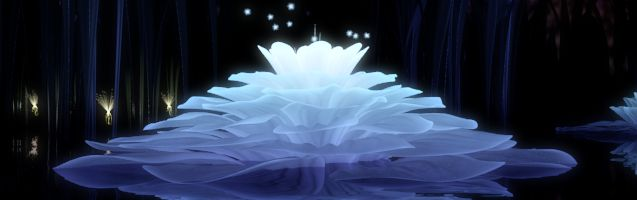## Introduction

Glow effects simulate the characteristics of a lens that suffers from light bleeding due to a high dynamic range. In photography, most cameras are set to use an average metering algorithm. The camera will analyze the frame and take the average exposure as the midpoint. Anything outside the camera's dynamic range will either become underexposed or overexposed. Due to the physics of camera lenses, overexposures can bleed into the image, giving off that glowish aura or whiteout if you're staring at a very bright source. This article will go over the process of how to produce these auras as well as examine some techniques for blending the result.

## The Technique

### First Pass

Rendering glows is done in two main stages. The first stage is to create what's called a glowmap. A glowmap is a texture object that contains only the emissive objects in the viewable area, but these objects must still be occluded by the non-emissive objects. A glowing lamp behind a wall should not be visible in the glowmap, for example. To do this, disable colour writes by calling gl.colorMask(false, false, false, false) and then draw all non-emissive geometry to the framebuffer object (FBO). The GPU will just write the depth values to the FBO. On the second pass, reenable colour writes and draw all emissive objects. You can render emissive objects with full lighting or you can disable lighting and render only the ambient/emissive lighting. It depends on the type of effect you're going for.

### Glowmap Resolution

Glowmaps don't need to use the same resolution as the window. It's best if you use a much lower resolution, typically in the range 64x64 to 256x256. At these lower resolutions, you don't need to use a large blurring kernel, which means you sample fewer pixels and thus improve performance. Glowmaps also don't need a whole lot of detail, so using a lower texture resolution is not that noticable.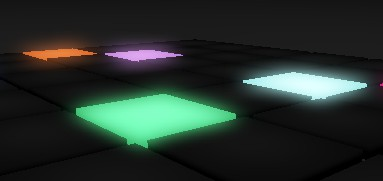Low resolution glowmap (128x128) with a 10 pixel blurring kernel.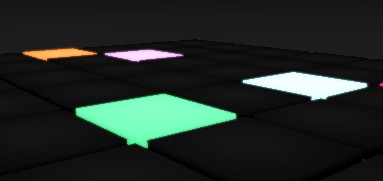High resolution glowmap (1024x1024) with a 10 pixel blurring kernel. Notice how the aura is practically invisible? This is because the blur kernel is to small for this resolution. To compensate for using a high resolution glowmap, you need to increase the kernel size to 30 - 40 pixels, which would significantly impact performance. One workaround for using such higher resolutions is to use a blur scale.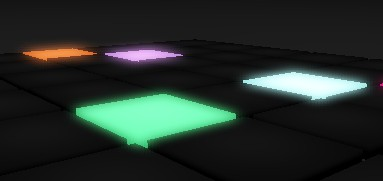The same high resolution glowmap with a 4x blur scale. When scaling the blur, you continue to sample with a 10 pixel blur kernel, but at each iteration you jump X number of pixels (called the blur scale). This will simulate using a larger kernel, but image quality will suffer if you use a large enough scale. You need to find the right balance, or simply stick to using a smaller resolution.

### Step 1: Render the Glowmap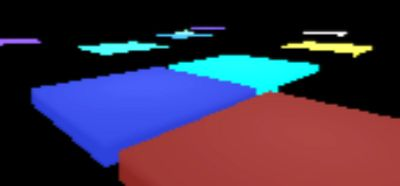Once you render the glowmap, blur it using a Gaussian blurring algorithm. This type of blurring will produce a nice smooth falloff the further away the blurred pixel is from the source.

### Step 2: Blur the Glowmap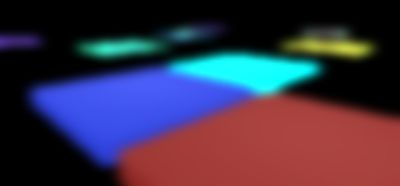Once you blur the glowmap, blend it with the fully rendered scene using some sort of overlay algorithm such as additive blending or screening. The blending operation you chose will have an effect on the result that is shown, so you need to play with the formulae and see what works best for you. Three blending operations are provided below.

### Step 3: Blend the Glowmap

src = glowmap

dst = rendered scene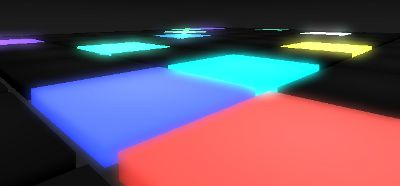pixel = src + dst

This is the easiest and fastest of the blending modes and is suitable for producing strong, overexposed auras.

Screen blending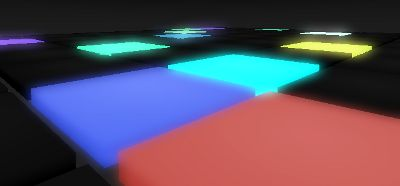pixel = (src + dst) - (src * dst)

This is the most common technique used for glows as it does not blowout an image like additive blending and it produces a nice subtle glow suitable for most applications.

Soft lighting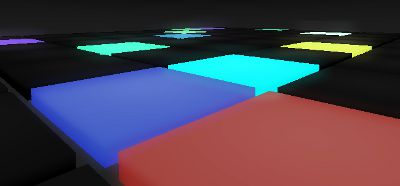$pixel = \left\{ \begin{matrix} dst - (1.0 - 2.0 * src) * dst * (1.0 - dst) & src \le 0.5 \\ dst + (2.0 * src - 1.0) * (4.0 * dst * (4.0 * dst + 1.0) * (dst - 1.0) + 7.0 * dst) & src \gt 0.5, dst \le 0.25 \\ dst + (2.0 * src - 1.0) * (\sqrt{dst} - dst) & src \gt 0.5, dst \gt 0.25 \end{matrix} \right\}$

This blending mode doesn't produce very strong auras, but the formula maintains highlights and creates a more vivid image. It is one of the better choices for dealing with luminescent objects like black lighting, glow in the dark stickers, and fiber optics to name a few examples. One thing to note about this formula is that it requires you have a neutral gray image, unlike the other two blending modes that require a black background. One quick way to do this in shader is to apply the following formula to the glowmap.

src = (src * 0.5) + 0.5

## Blurring

The separable blurring technique (also known as the box-blur) gets its name from the way it performs blurring. In the traditional sense, blurring is performed using a convolution filter. That is, an N x M matrix that samples neighbouring pixels and finds an average. A faster way to perform this activity is to separate the blurring into two passes. The first pass will blur all pixels horizontally. The second pass will blur all pixels vertically. The result is the same as performing blur with a convolution filter at a significantly faster speed.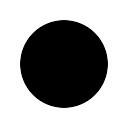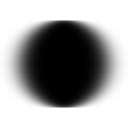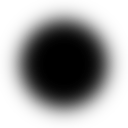Left: Unfiltered image.

Middle: Pass 1, horizontal blurring applied.

Right: Pass 2, vertical blurring applied.

What's unique here is that the lower resolution you use, the more effective the blurring. A 256x256 texture for instance can produce a very soft blur with a small blur radius, whereas a 1024x1024 texture requires a large kernel to blur it sufficiently enough. You have to find the right balance between resolution and blurring. To much of either can hinder performance. Glowmaps tend to work best with lower resolutions, between 64x64 and 256x256.

### Gaussian Filter

The Gaussian blur filter produces a smooth falloff, which works very well with glowmaps. Since we're performing the blur in two steps, one for the X-axis and another for the Y-axis, we only need to use a 1-dimensional Gaussian filter.

$\frac{1.0}{\sqrt{2\pi * \sigma}} * e^{\frac{-x^2}{2\sigma}}$

Where

x is the positive or negative offset from the sample point. The sample point is located at x = 0.

$\sigma$ is the standard deviation, which controls how much the blur deviates from the source pixel. The greater the deviation, the more faded and less intense the blur appears. The smaller the deviation, the more intense or blocky the blur will appear. The demo uses 1/6th the blur radius, which produces good results.

### Blur Strength

The Gaussian formula produces a more intense value the closer you are to the sample point. That is, the closer x is to 0. Typically for every 1 pixel you advance in the texture, you advance 1 unit in the Gaussian formula. If you multiply the Gaussian offset by a value less than 1.0, you will reduce the falloff rate of the blur and produce a more overexposed result. This can be useful when you want to intensify the aura for a specific purpose. This adjusts the Gaussian formula such that:

x = x * (1.0 - strength)

Where

$0 < strength \le 1$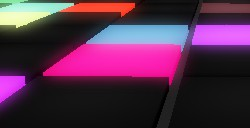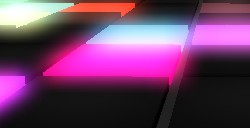Sampling at one-pixel intervals, the image on the left shows a Gaussian filter with a blur strength of 0 and the image on the right shows a blur strength of 0.4.

### Extra: Image Softening

Since the glowmap uses blurring to produce auras, the same technique is also used to soften an image.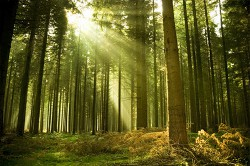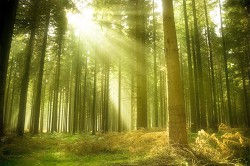The image on the left shows a standard photograph, but the image on the right shows what happens when you apply the same technique used for glowmaps. You pass the entire rendered frame into the blur shader and then screen the result on top of the original frame. The end result is a softer, more dreamy looking image.

## Conclusion

Glow effects have a wide variety of uses and improve the image quality substantially, even if the effect is used superficially. Particle effects, light coronas, neon lights, luminescent objects, image softening are just some examples of where glow effects shine. Glowmaps are easy to implement and fast to render, making them a useful feature for any developer on any platform.

The source code for this project is made freely available for download. The ZIP package below contains both the HTML and JavaScript files to replicate this WebGL demo.

The source code utilizes the Nutty Open WebGL Framework, which is an open sourced, simplified version of the closed source Nutty WebGL Framework. It is released under a modified MIT license, so you are free to use if for personal and commercial purposes.